# Soal Fisika A Carnot Engine Uses High Temperature Reservoir

1. A Carnot engine uses a high temperature reservoir with temperature 800 K and efficiency 40%. In order to increase the efficiency to 50%, the temperature of the high reservoir must be increased to

T1 = 800 K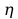= 40%= 50%

T1= ?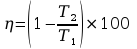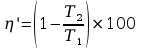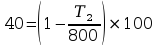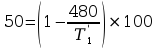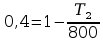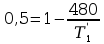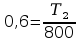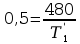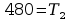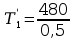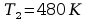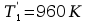1. Efficiency of Carnot engine that operated with high temperature 400 K is 40 %, if the high temperature increase to 640 K. Calculate the efficiency!= 40%

T1 = 400 K

T1 = 640 K

= ?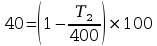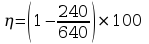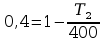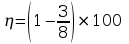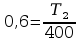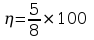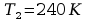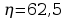1. Look at to a gas P-V graphic below :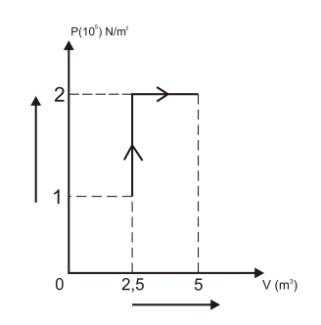The ideal gas during A-B process. Gas work during AB process is

1. Much work is done 1mol of gas if the gas isotermic expansion of 2 liters volume at pressures up 20.105 Nm-2 to 20 liters (the gas temperature 270C) is

2. On the graph below calculate the work done by machine!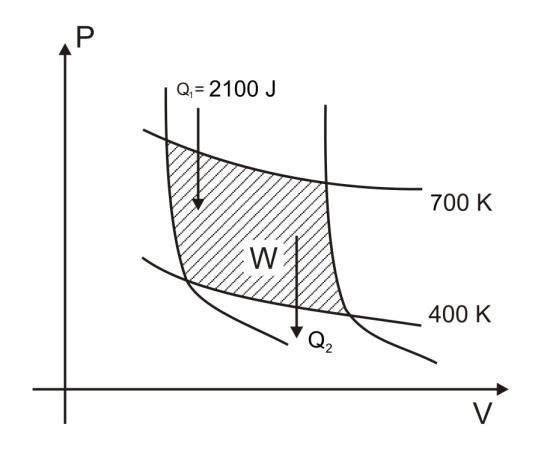Q1= 2100 J

T1 = 700K

T2 = 400 K

W =.?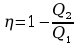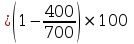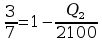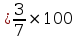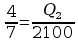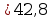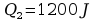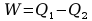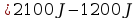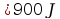1. An ideal refrigerator using 275 watts of electrical power and be able to remove the heat out of the machine at a rate of 850 joules per second. If the temperature inside the refrigerator 370C, determine the temperature outside the refrigerator!

W = 275 Watt

Q1 = 850 Joule

T2 = 370C = 3100K

T1 = .?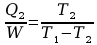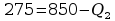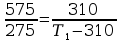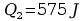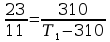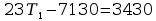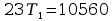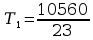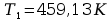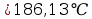1. A Carnot engine uses a high temperature reservoir with temperature 300 K have efficiency 40%. If the high temperature reservoir have temperature 360 K, the efficiency become.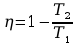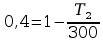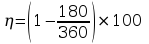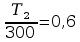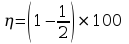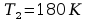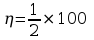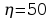1. Gases with internal energy 60 J absorb a heat 90 J from environment. If in the same time, system get work 150 J. Determine the internal energy at last!

U1 = 60 J

Q = 90 J

W = -150 J

U2 = ?

U = Q W

U2 U1 = Q W

U2 60 = 90 (-150)

U2 = 240 + 60

U2 = 300 J

1. If R = 8,31 J/mol.K, so heat capacity of 4 mol nitrogen gases in constant pressure and low temperature is.

2. Heat capacity of 5 mol monoatomic gases in constant volume is.(R = 8,31 J/mol.K)